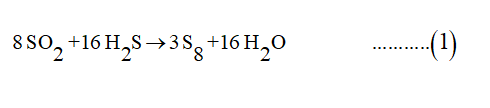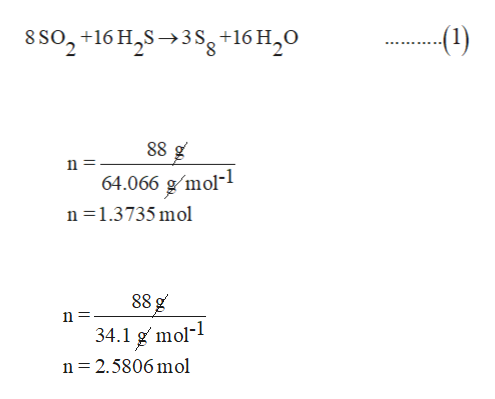# What is the maximum mass of S8S8 that can be produced by combining 88.0 g88.0 g of each reactant? 8SO2+16H2S⟶3S8+16H2O mass of S8:

Question

What is the maximum mass of S8S8 that can be produced by combining 88.0 g88.0 g of each reactant?

8SO2+16H2S⟶3S8+16H2O

mass of S8:

check_circleExpert Solution
Step 1

According to the given chemical reaction, S8 molecules are produced when SO2 reacts with H2S.

The mass of both reactants is 88 g each.

The molecular mass of SO2 =64.066 g mol-1

The molecular mass of H2S = 34.1 g mol-1

Since no.of mole is given mass per molecular mass.

So no. of mole (n) of SO2 -:

No. of moles of H2S -:help_outlineImage Transcriptionclose8 So2+16 H2S3 sg+16 H20 -(1) 88 g 64.066 gmol n 1.3735 mol 88 g n= mol- 34.1 n 2.5806 mol fullscreen
Step 2

According to given equation (1), the stoichiometric ratio of SO2 and H2S is 1:2, which means 1 mole of  SO2 reacts with 2moles of H2S.

But from our calculation 1.3735 moles of SO2 reacts with 2.5806 moles of  H2S.

Converting it into simple rat...

### Want to see the full answer?

See Solution

#### Want to see this answer and more?

Solutions are written by subject experts who are available 24/7. Questions are typically answered within 1 hour*

See Solution
*Response times may vary by subject and question
Tagged in

### Chemistry